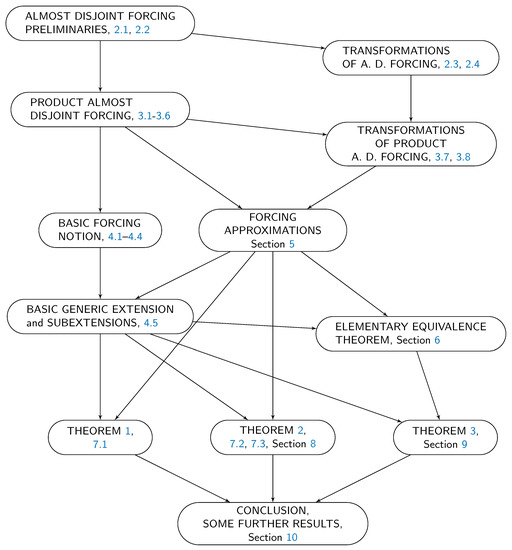Previous Article in Journal
Construction of Different Types Analytic Solutions for the Zhiber-Shabat Equation
Open AccessArticle

# Models of Set Theory in which Nonconstructible Reals First Appear at a Given Projective Level

byVladimir Kanovei *,†andVassily Lyubetsky
Institute for Information Transmission Problems of the Russian Academy of Sciences, 127051 Moscow, Russia
*
Author to whom correspondence should be addressed.
These authors contributed equally to this work.
Mathematics 2020, 8(6), 910; https://doi.org/10.3390/math8060910
Received: 11 May 2020 / Revised: 25 May 2020 / Accepted: 27 May 2020 / Published: 3 June 2020
(This article belongs to the Special Issue Mathematical Logic and Its Applications 2020)
Models of set theory are defined, in which nonconstructible reals first appear on a given level of the projective hierarchy. Our main results are as follows. Suppose that $n ≥ 2$ . Then: 1. If it holds in the constructible universe $L$ that $a ⊆ ω$ and $a ∉ Σ n 1 ∪ Π n 1$ , then there is a generic extension of $L$ in which $a ∈ Δ n + 1 1$ but still $a ∉ Σ n 1 ∪ Π n 1$ , and moreover, any set $x ⊆ ω$ , $x ∈ Σ n 1$ , is constructible and $Σ n 1$ in $L$ . 2. There exists a generic extension $L$ in which it is true that there is a nonconstructible $Δ n + 1 1$ set $a ⊆ ω$ , but all $Σ n 1$ sets $x ⊆ ω$ are constructible and even $Σ n 1$ in $L$ , and in addition, $V = L [ a ]$ in the extension. 3. There exists an generic extension of $L$ in which there is a nonconstructible $Σ n + 1 1$ set $a ⊆ ω$ , but all $Δ n + 1 1$ sets $x ⊆ ω$ are constructible and $Δ n + 1 1$ in $L$ . Thus, nonconstructible reals (here subsets of $ω$ ) can first appear at a given lightface projective class strictly higher than $Σ 2 1$ , in an appropriate generic extension of $L$ . The lower limit $Σ 2 1$ is motivated by the Shoenfield absoluteness theorem, which implies that all $Σ 2 1$ sets $a ⊆ ω$ are constructible. Our methods are based on almost-disjoint forcing. We add a sufficient number of generic reals to $L$ , which are very similar at a given projective level n but discernible at the next level $n + 1$ . View Full-Text
Show FiguresFigure 1

MDPI and ACS Style

Kanovei, V.; Lyubetsky, V. Models of Set Theory in which Nonconstructible Reals First Appear at a Given Projective Level. Mathematics 2020, 8, 910.